# Fractions - math word problems

1. Jose and Kaitlyn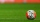Jose and Kaitlyn have a contest to see who can throw a baseball the farthest. Kaitlyn wins, with a throw of 200 ft. If Jose threw the ball 3/4 as far as Kaitlyn, how far did Jose throw the ball?
2. BoatsThree-quarters of boats are white, 1/7 are blue and 9 are red. How many boats do we have?
3. Water reservoir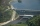One drain hole emptied the water from the tank for 20 hours, with the second drain for 30 hours. How long will the water drain from this tank if we open two drains simultaneously?
4. Equivalent expressionsA coach took his team out for pizza after their last game. There were 14 players, so they had to sit in smaller groups at different tables. Six players sat at one table and got 4 small pizzas to share equally. The other players sat at the different table
5. What is one third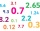What is 1/3 as a decimal? Give your answer rounded to 2 decimal places.
6. Honza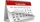Honza is 13 years old and Peter 21 years old. After how many years are their ages will be at ratio 7: 9?
7. BucketThe bucket half filled with water weighs 5.55 kg, the full bucket weighs 9.85 kg. How much does the bucket weigh?
8. What is 3What is the least common denominator of 11/15 and 12/19?
9. Degrees Fahrenheit? The equation above shows how temperature F, measured in degrees Fahrenheit, relates to a temperature C, measured in degrees Celsius. Based on the equation, which of the following must be true? I. A temperature increase of 1 degree Fahrenheit is equi
10. Unknown numberI think the number. I'll reduce it to its one-third. The result is then increased by one-third, and I get the number 12.
11. EmployeesOf all 360 employees, there are 11/12 women. How many men work in a company?
12. Write 2Write 791 thousandths as fraction in expanded form.
13. The Mayflower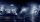The Mayflower traveled for 66 days on the trip from England to America. The weather was storming for many days of their trip. If one and a half of the days at Sea where Sunny with good weather, 1/6 of the days were sunny but very windy and the other days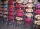Cris had 15000 . He spent 1/3 of his money on a table and the remaining on 5 similar chairs that cost the same. How much did one chair cost?
15. Soup from canteen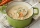For how many people is 90 liters of soup enough if we assume 3/8 liter of soup per person in the canteen?
16. The thirdThe one-third rod is blue, one-half of the rod is red, the rest of the rod is white and measures 8 cm. How long is the whole rod?
17. Equation 20In given equation: 8/9-4/5=2/9+x, find x
18. The perimeter 2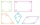The perimeter of the quadrilateral a = 1m b = 14/5m c = 2 3/10m d = 1 4/5m?
19. Winery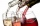The wine processing plant sold 8/15 of its total wine production to the domestic market, 2/7 to the foreign the rest of the wine. How many percents are still in stock?
20. Watching TVOne evening 2/3 students watch TV. Of those students, 3/8 watched a reality show. Of the students that watched the show, 1/4 of them recorded it. What fraction of the students watched and recorded reality tv.

Do you have an interesting mathematical word problem that you can't solve it? Submit math problem, and we can try to solve it.

We will send a solution to your e-mail address. Solved examples are also published here. Please enter the e-mail correctly and check whether you don't have a full mailbox.

Please do not submit problems from current active competitions such as Mathematical Olympiad, correspondence seminars etc...

Need help calculate sum, simplify or multiply fractions? Try our fraction calculator.